# Module: color¶

 Convert an image array to a new color space. Make an educated guess about whether an image has a channels dimension. skimage.color.rgba2rgb(rgba[, background]) RGBA to RGB conversion. RGB to HSV color space conversion. HSV to RGB color space conversion. RGB to XYZ color space conversion. XYZ to RGB color space conversion. RGB to RGB CIE color space conversion. RGB CIE to RGB color space conversion. Compute luminance of an RGB image. Compute luminance of an RGB image. skimage.color.gray2rgb(image[, alpha]) Create an RGB representation of a gray-level image. skimage.color.grey2rgb(image[, alpha]) Create an RGB representation of a gray-level image. skimage.color.xyz2lab(xyz[, illuminant, …]) XYZ to CIE-LAB color space conversion. skimage.color.lab2xyz(lab[, illuminant, …]) CIE-LAB to XYZcolor space conversion. skimage.color.lab2rgb(lab[, illuminant, …]) Lab to RGB color space conversion. skimage.color.rgb2lab(rgb[, illuminant, …]) RGB to lab color space conversion. RGB to Haematoxylin-Eosin-DAB (HED) color space conversion. Haematoxylin-Eosin-DAB (HED) to RGB color space conversion. CIE-LAB to CIE-LCH color space conversion. CIE-LCH to CIE-LAB color space conversion. RGB to YUV color space conversion. YUV to RGB color space conversion. RGB to YIQ color space conversion. YIQ to RGB color space conversion. RGB to YPbPr color space conversion. YPbPr to RGB color space conversion. RGB to YCbCr color space conversion. YCbCr to RGB color space conversion. RGB to YDbDr color space conversion. YDbDr to RGB color space conversion. skimage.color.separate_stains(rgb, conv_matrix) RGB to stain color space conversion. skimage.color.combine_stains(stains, conv_matrix) Stain to RGB color space conversion. skimage.color.label2rgb(label[, image, …]) Return an RGB image where color-coded labels are painted over the image. skimage.color.deltaE_cie76(lab1, lab2) Euclidean distance between two points in Lab color space skimage.color.deltaE_ciede94(lab1, lab2[, …]) Color difference according to CIEDE 94 standard skimage.color.deltaE_ciede2000(lab1, lab2[, …]) Color difference as given by the CIEDE 2000 standard. skimage.color.deltaE_cmc(lab1, lab2[, kL, kC]) Color difference from the CMC l:c standard.

## convert_colorspace¶

skimage.color.convert_colorspace(arr, fromspace, tospace)[source]

Convert an image array to a new color space.

Valid color spaces are:

‘RGB’, ‘HSV’, ‘RGB CIE’, ‘XYZ’, ‘YUV’, ‘YIQ’, ‘YPbPr’, ‘YCbCr’, ‘YDbDr’

Parameters
arrarray_like

The image to convert.

fromspacevalid color space

The color space to convert from. Can be specified in lower case.

tospacevalid color space

The color space to convert to. Can be specified in lower case.

Returns
outndarray

The converted image.

Notes

Conversion is performed through the “central” RGB color space, i.e. conversion from XYZ to HSV is implemented as XYZ -> RGB -> HSV instead of directly.

Examples

>>> from skimage import data
>>> img = data.astronaut()
>>> img_hsv = convert_colorspace(img, 'RGB', 'HSV')


## guess_spatial_dimensions¶

skimage.color.guess_spatial_dimensions(image)[source]

Make an educated guess about whether an image has a channels dimension.

Parameters
imagendarray

The input image.

Returns
spatial_dimsint or None

The number of spatial dimensions of image. If ambiguous, the value is None.

Raises
ValueError

If the image array has less than two or more than four dimensions.

## rgba2rgb¶

skimage.color.rgba2rgb(rgba, background=(1, 1, 1))[source]

RGBA to RGB conversion.

Parameters
rgbaarray_like

The image in RGBA format, in a 3-D array of shape (.., .., 4).

backgroundarray_like

The color of the background to blend the image with. A tuple containing 3 floats between 0 to 1 - the RGB value of the background.

Returns
outndarray

The image in RGB format, in a 3-D array of shape (.., .., 3).

Raises
ValueError

If rgba is not a 3-D array of shape (.., .., 4).

References

1

https://en.wikipedia.org/wiki/Alpha_compositing#Alpha_blending

Examples

>>> from skimage import color
>>> from skimage import data
>>> img_rgba = data.logo()
>>> img_rgb = color.rgba2rgb(img_rgba)


## rgb2hsv¶

skimage.color.rgb2hsv(rgb)[source]

RGB to HSV color space conversion.

Parameters
rgbarray_like

The image in RGB format, in a 3-D array of shape (.., .., 3).

Returns
outndarray

The image in HSV format, in a 3-D array of shape (.., .., 3).

Raises
ValueError

If rgb is not a 3-D array of shape (.., .., 3).

Notes

Conversion between RGB and HSV color spaces results in some loss of precision, due to integer arithmetic and rounding .

References

1

https://en.wikipedia.org/wiki/HSL_and_HSV

Examples

>>> from skimage import color
>>> from skimage import data
>>> img = data.astronaut()
>>> img_hsv = color.rgb2hsv(img)


### Examples using skimage.color.rgb2hsv¶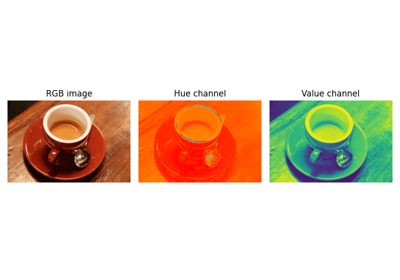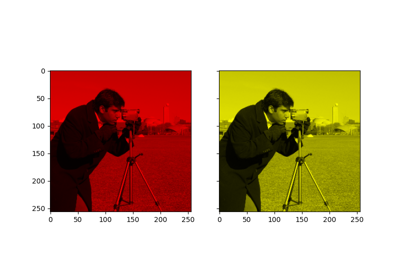## hsv2rgb¶

skimage.color.hsv2rgb(hsv)[source]

HSV to RGB color space conversion.

Parameters
hsvarray_like

The image in HSV format, in a 3-D array of shape (.., .., 3).

Returns
outndarray

The image in RGB format, in a 3-D array of shape (.., .., 3).

Raises
ValueError

If hsv is not a 3-D array of shape (.., .., 3).

Notes

Conversion between RGB and HSV color spaces results in some loss of precision, due to integer arithmetic and rounding .

References

1

https://en.wikipedia.org/wiki/HSL_and_HSV

Examples

>>> from skimage import data
>>> img = data.astronaut()
>>> img_hsv = rgb2hsv(img)
>>> img_rgb = hsv2rgb(img_hsv)


### Examples using skimage.color.hsv2rgb¶## rgb2xyz¶

skimage.color.rgb2xyz(rgb)[source]

RGB to XYZ color space conversion.

Parameters
rgbarray_like

The image in RGB format, in a 3- or 4-D array of shape (.., ..,[ ..,] 3).

Returns
outndarray

The image in XYZ format, in a 3- or 4-D array of shape (.., ..,[ ..,] 3).

Raises
ValueError

If rgb is not a 3- or 4-D array of shape (.., ..,[ ..,] 3).

Notes

The CIE XYZ color space is derived from the CIE RGB color space. Note however that this function converts from sRGB.

References

1

https://en.wikipedia.org/wiki/CIE_1931_color_space

Examples

>>> from skimage import data
>>> img = data.astronaut()
>>> img_xyz = rgb2xyz(img)


## xyz2rgb¶

skimage.color.xyz2rgb(xyz)[source]

XYZ to RGB color space conversion.

Parameters
xyzarray_like

The image in XYZ format, in a 3-D array of shape (.., .., 3).

Returns
outndarray

The image in RGB format, in a 3-D array of shape (.., .., 3).

Raises
ValueError

If xyz is not a 3-D array of shape (.., .., 3).

Notes

The CIE XYZ color space is derived from the CIE RGB color space. Note however that this function converts to sRGB.

References

1

https://en.wikipedia.org/wiki/CIE_1931_color_space

Examples

>>> from skimage import data
>>> from skimage.color import rgb2xyz, xyz2rgb
>>> img = data.astronaut()
>>> img_xyz = rgb2xyz(img)
>>> img_rgb = xyz2rgb(img_xyz)


## rgb2rgbcie¶

skimage.color.rgb2rgbcie(rgb)[source]

RGB to RGB CIE color space conversion.

Parameters
rgbarray_like

The image in RGB format, in a 3-D array of shape (.., .., 3).

Returns
outndarray

The image in RGB CIE format, in a 3-D array of shape (.., .., 3).

Raises
ValueError

If rgb is not a 3-D array of shape (.., .., 3).

References

1

https://en.wikipedia.org/wiki/CIE_1931_color_space

Examples

>>> from skimage import data
>>> from skimage.color import rgb2rgbcie
>>> img = data.astronaut()
>>> img_rgbcie = rgb2rgbcie(img)


## rgbcie2rgb¶

skimage.color.rgbcie2rgb(rgbcie)[source]

RGB CIE to RGB color space conversion.

Parameters
rgbciearray_like

The image in RGB CIE format, in a 3-D array of shape (.., .., 3).

Returns
outndarray

The image in RGB format, in a 3-D array of shape (.., .., 3).

Raises
ValueError

If rgbcie is not a 3-D array of shape (.., .., 3).

References

1

https://en.wikipedia.org/wiki/CIE_1931_color_space

Examples

>>> from skimage import data
>>> from skimage.color import rgb2rgbcie, rgbcie2rgb
>>> img = data.astronaut()
>>> img_rgbcie = rgb2rgbcie(img)
>>> img_rgb = rgbcie2rgb(img_rgbcie)


## rgb2grey¶

skimage.color.rgb2grey(rgb)[source]

Compute luminance of an RGB image.

Parameters
rgbarray_like

The image in RGB format, in a 3-D or 4-D array of shape (.., ..,[ ..,] 3), or in RGBA format with shape (.., ..,[ ..,] 4).

Returns
outndarray

The luminance image - an array which is the same size as the input array, but with the channel dimension removed.

Raises
ValueError

If rgb2gray is not a 3-D or 4-D arrays of shape (.., ..,[ ..,] 3) or (.., ..,[ ..,] 4).

Notes

The weights used in this conversion are calibrated for contemporary CRT phosphors:

Y = 0.2125 R + 0.7154 G + 0.0721 B


If there is an alpha channel present, it is ignored.

References

1

http://www.poynton.com/PDFs/ColorFAQ.pdf

Examples

>>> from skimage.color import rgb2gray
>>> from skimage import data
>>> img = data.astronaut()
>>> img_gray = rgb2gray(img)


## rgb2gray¶

skimage.color.rgb2gray(rgb)[source]

Compute luminance of an RGB image.

Parameters
rgbarray_like

The image in RGB format, in a 3-D or 4-D array of shape (.., ..,[ ..,] 3), or in RGBA format with shape (.., ..,[ ..,] 4).

Returns
outndarray

The luminance image - an array which is the same size as the input array, but with the channel dimension removed.

Raises
ValueError

If rgb2gray is not a 3-D or 4-D arrays of shape (.., ..,[ ..,] 3) or (.., ..,[ ..,] 4).

Notes

The weights used in this conversion are calibrated for contemporary CRT phosphors:

Y = 0.2125 R + 0.7154 G + 0.0721 B


If there is an alpha channel present, it is ignored.

References

1

http://www.poynton.com/PDFs/ColorFAQ.pdf

Examples

>>> from skimage.color import rgb2gray
>>> from skimage import data
>>> img = data.astronaut()
>>> img_gray = rgb2gray(img)


### Examples using skimage.color.rgb2gray¶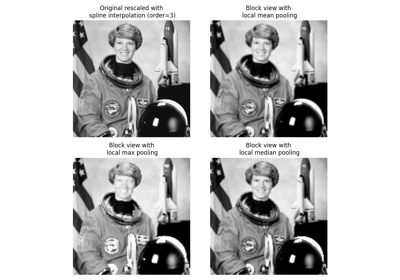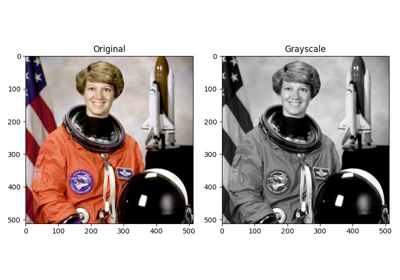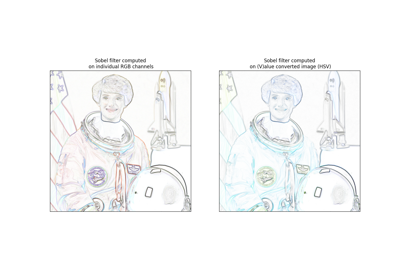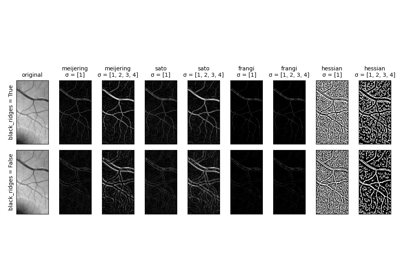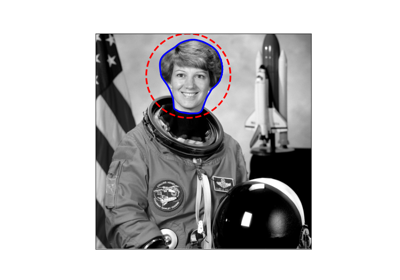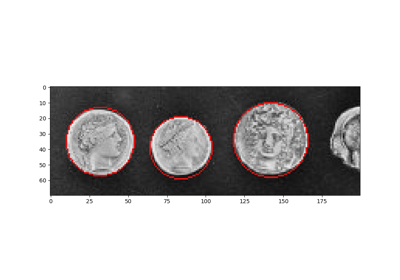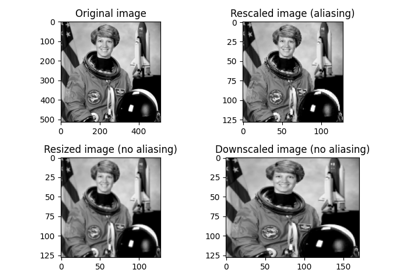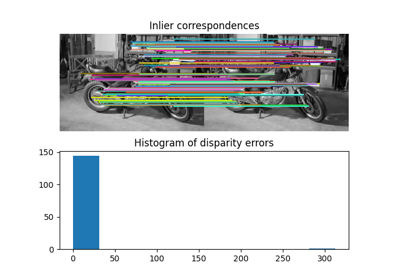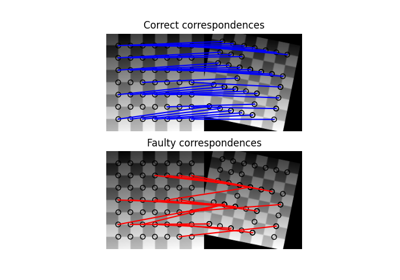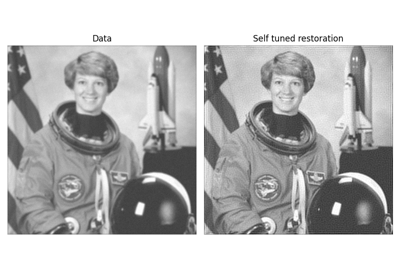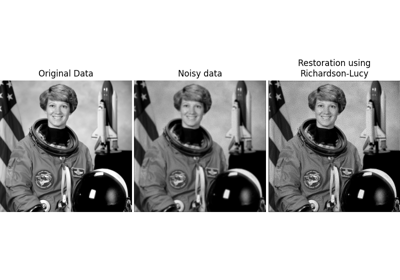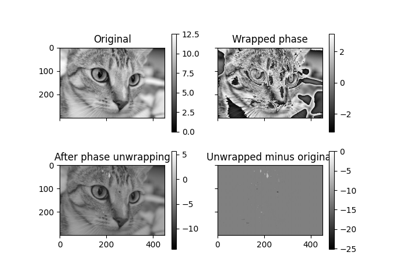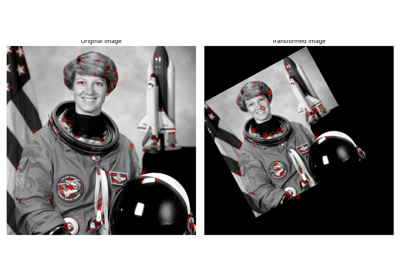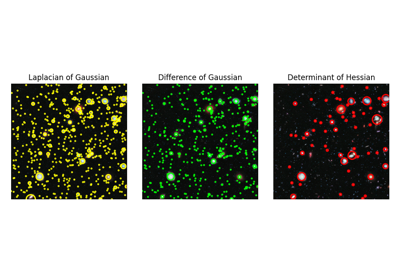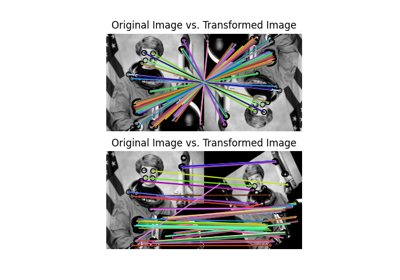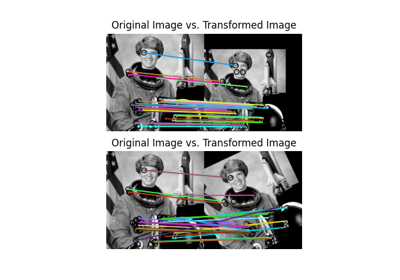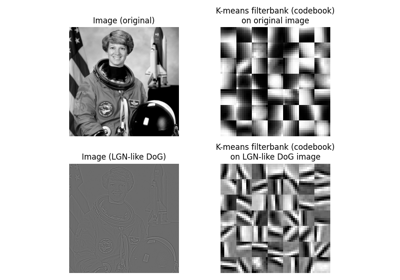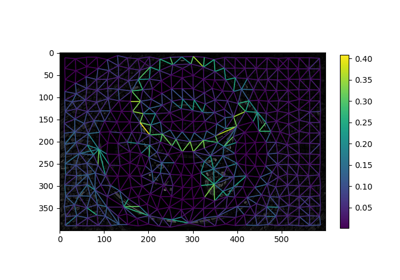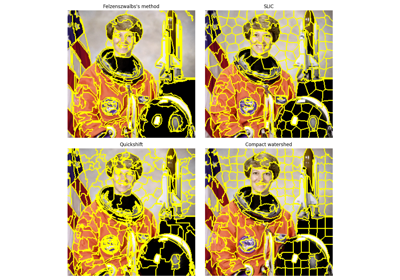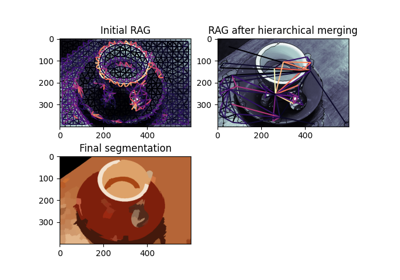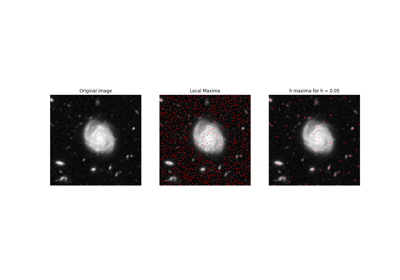## gray2rgb¶

skimage.color.gray2rgb(image, alpha=None)[source]

Create an RGB representation of a gray-level image.

Parameters
imagearray_like

Input image of shape (M[, N][, P]).

alphabool, optional

Ensure that the output image has an alpha layer. If None, alpha layers are passed through but not created.

Returns
rgbndarray

RGB image of shape (M[, N][, P], 3).

Raises
ValueError

If the input is not a 1-, 2- or 3-dimensional image.

Notes

If the input is a 1-dimensional image of shape (M, ), the output will be shape (M, 3).

### Examples using skimage.color.gray2rgb¶## grey2rgb¶

skimage.color.grey2rgb(image, alpha=None)[source]

Create an RGB representation of a gray-level image.

Parameters
imagearray_like

Input image of shape (M[, N][, P]).

alphabool, optional

Ensure that the output image has an alpha layer. If None, alpha layers are passed through but not created.

Returns
rgbndarray

RGB image of shape (M[, N][, P], 3).

Raises
ValueError

If the input is not a 1-, 2- or 3-dimensional image.

Notes

If the input is a 1-dimensional image of shape (M, ), the output will be shape (M, 3).

## xyz2lab¶

skimage.color.xyz2lab(xyz, illuminant='D65', observer='2')[source]

XYZ to CIE-LAB color space conversion.

Parameters
xyzarray_like

The image in XYZ format, in a 3- or 4-D array of shape (.., ..,[ ..,] 3).

illuminant{“A”, “D50”, “D55”, “D65”, “D75”, “E”}, optional

The name of the illuminant (the function is NOT case sensitive).

observer{“2”, “10”}, optional

The aperture angle of the observer.

Returns
outndarray

The image in CIE-LAB format, in a 3- or 4-D array of shape (.., ..,[ ..,] 3).

Raises
ValueError

If xyz is not a 3-D array of shape (.., ..,[ ..,] 3).

ValueError

If either the illuminant or the observer angle is unsupported or unknown.

Notes

By default Observer= 2A, Illuminant= D65. CIE XYZ tristimulus values x_ref=95.047, y_ref=100., z_ref=108.883. See function get_xyz_coords for a list of supported illuminants.

References

1

http://www.easyrgb.com/index.php?X=MATH&H=07#text7

2

https://en.wikipedia.org/wiki/Lab_color_space

Examples

>>> from skimage import data
>>> from skimage.color import rgb2xyz, xyz2lab
>>> img = data.astronaut()
>>> img_xyz = rgb2xyz(img)
>>> img_lab = xyz2lab(img_xyz)


## lab2xyz¶

skimage.color.lab2xyz(lab, illuminant='D65', observer='2')[source]

CIE-LAB to XYZcolor space conversion.

Parameters
labarray_like

The image in lab format, in a 3-D array of shape (.., .., 3).

illuminant{“A”, “D50”, “D55”, “D65”, “D75”, “E”}, optional

The name of the illuminant (the function is NOT case sensitive).

observer{“2”, “10”}, optional

The aperture angle of the observer.

Returns
outndarray

The image in XYZ format, in a 3-D array of shape (.., .., 3).

Raises
ValueError

If lab is not a 3-D array of shape (.., .., 3).

ValueError

If either the illuminant or the observer angle are not supported or unknown.

UserWarning

If any of the pixels are invalid (Z < 0).

Notes

By default Observer= 2A, Illuminant= D65. CIE XYZ tristimulus values x_ref = 95.047, y_ref = 100., z_ref = 108.883. See function ‘get_xyz_coords’ for a list of supported illuminants.

References

1

http://www.easyrgb.com/index.php?X=MATH&H=07#text7

2

https://en.wikipedia.org/wiki/Lab_color_space

## lab2rgb¶

skimage.color.lab2rgb(lab, illuminant='D65', observer='2')[source]

Lab to RGB color space conversion.

Parameters
labarray_like

The image in Lab format, in a 3-D array of shape (.., .., 3).

illuminant{“A”, “D50”, “D55”, “D65”, “D75”, “E”}, optional

The name of the illuminant (the function is NOT case sensitive).

observer{“2”, “10”}, optional

The aperture angle of the observer.

Returns
outndarray

The image in RGB format, in a 3-D array of shape (.., .., 3).

Raises
ValueError

If lab is not a 3-D array of shape (.., .., 3).

Notes

This function uses lab2xyz and xyz2rgb. By default Observer= 2A, Illuminant= D65. CIE XYZ tristimulus values x_ref=95.047, y_ref=100., z_ref=108.883. See function get_xyz_coords for a list of supported illuminants.

References

1

https://en.wikipedia.org/wiki/Standard_illuminant

## rgb2lab¶

skimage.color.rgb2lab(rgb, illuminant='D65', observer='2')[source]

RGB to lab color space conversion.

Parameters
rgbarray_like

The image in RGB format, in a 3- or 4-D array of shape (.., ..,[ ..,] 3).

illuminant{“A”, “D50”, “D55”, “D65”, “D75”, “E”}, optional

The name of the illuminant (the function is NOT case sensitive).

observer{“2”, “10”}, optional

The aperture angle of the observer.

Returns
outndarray

The image in Lab format, in a 3- or 4-D array of shape (.., ..,[ ..,] 3).

Raises
ValueError

If rgb is not a 3- or 4-D array of shape (.., ..,[ ..,] 3).

Notes

This function uses rgb2xyz and xyz2lab. By default Observer= 2A, Illuminant= D65. CIE XYZ tristimulus values x_ref=95.047, y_ref=100., z_ref=108.883. See function get_xyz_coords for a list of supported illuminants.

References

1

https://en.wikipedia.org/wiki/Standard_illuminant

## rgb2hed¶

skimage.color.rgb2hed(rgb)[source]

RGB to Haematoxylin-Eosin-DAB (HED) color space conversion.

Parameters
rgbarray_like

The image in RGB format, in a 3-D array of shape (.., .., 3).

Returns
outndarray

The image in HED format, in a 3-D array of shape (.., .., 3).

Raises
ValueError

If rgb is not a 3-D array of shape (.., .., 3).

References

1

A. C. Ruifrok and D. A. Johnston, “Quantification of histochemical staining by color deconvolution.,” Analytical and quantitative cytology and histology / the International Academy of Cytology [and] American Society of Cytology, vol. 23, no. 4, pp. 291-9, Aug. 2001.

Examples

>>> from skimage import data
>>> from skimage.color import rgb2hed
>>> ihc = data.immunohistochemistry()
>>> ihc_hed = rgb2hed(ihc)


### Examples using skimage.color.rgb2hed¶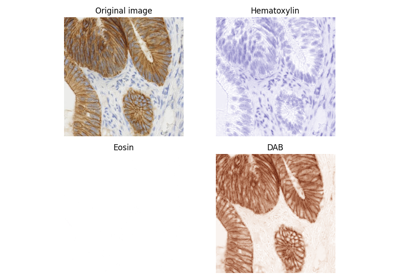## hed2rgb¶

skimage.color.hed2rgb(hed)[source]

Haematoxylin-Eosin-DAB (HED) to RGB color space conversion.

Parameters
hedarray_like

The image in the HED color space, in a 3-D array of shape (.., .., 3).

Returns
outndarray

The image in RGB, in a 3-D array of shape (.., .., 3).

Raises
ValueError

If hed is not a 3-D array of shape (.., .., 3).

References

1

A. C. Ruifrok and D. A. Johnston, “Quantification of histochemical staining by color deconvolution.,” Analytical and quantitative cytology and histology / the International Academy of Cytology [and] American Society of Cytology, vol. 23, no. 4, pp. 291-9, Aug. 2001.

Examples

>>> from skimage import data
>>> from skimage.color import rgb2hed, hed2rgb
>>> ihc = data.immunohistochemistry()
>>> ihc_hed = rgb2hed(ihc)
>>> ihc_rgb = hed2rgb(ihc_hed)


## lab2lch¶

skimage.color.lab2lch(lab)[source]

CIE-LAB to CIE-LCH color space conversion.

LCH is the cylindrical representation of the LAB (Cartesian) colorspace

Parameters
labarray_like

The N-D image in CIE-LAB format. The last (N+1-th) dimension must have at least 3 elements, corresponding to the L, a, and b color channels. Subsequent elements are copied.

Returns
outndarray

The image in LCH format, in a N-D array with same shape as input lab.

Raises
ValueError

If lch does not have at least 3 color channels (i.e. l, a, b).

Notes

The Hue is expressed as an angle between (0, 2*pi)

Examples

>>> from skimage import data
>>> from skimage.color import rgb2lab, lab2lch
>>> img = data.astronaut()
>>> img_lab = rgb2lab(img)
>>> img_lch = lab2lch(img_lab)


## lch2lab¶

skimage.color.lch2lab(lch)[source]

CIE-LCH to CIE-LAB color space conversion.

LCH is the cylindrical representation of the LAB (Cartesian) colorspace

Parameters
lcharray_like

The N-D image in CIE-LCH format. The last (N+1-th) dimension must have at least 3 elements, corresponding to the L, a, and b color channels. Subsequent elements are copied.

Returns
outndarray

The image in LAB format, with same shape as input lch.

Raises
ValueError

If lch does not have at least 3 color channels (i.e. l, c, h).

Examples

>>> from skimage import data
>>> from skimage.color import rgb2lab, lch2lab
>>> img = data.astronaut()
>>> img_lab = rgb2lab(img)
>>> img_lch = lab2lch(img_lab)
>>> img_lab2 = lch2lab(img_lch)


## rgb2yuv¶

skimage.color.rgb2yuv(rgb)[source]

RGB to YUV color space conversion.

Parameters
rgbarray_like

The image in RGB format, in a 3- or 4-D array of shape (M, N, [P,] 3).

Returns
outndarray

The image in YUV format, in a 3- or 4-D array of shape (M, N, [P,] 3).

Raises
ValueError

If rgb is not a 3- or 4-D array of shape (M, N, [P,] 3).

Notes

Y is between 0 and 1. Use YCbCr instead of YUV for the color space which is commonly used by video codecs (where Y ranges from 16 to 235)

References

1

https://en.wikipedia.org/wiki/YUV

## yuv2rgb¶

skimage.color.yuv2rgb(yuv)[source]

YUV to RGB color space conversion.

Parameters
yuvarray_like

The image in YUV format, in a 3- or 4-D array of shape (M, N, [P,] 3).

Returns
outndarray

The image in RGB format, in a 3- or 4-D array of shape (M, N, [P,] 3).

Raises
ValueError

If yuv is not a 3- or 4-D array of shape (M, N, [P,] 3).

References

1

https://en.wikipedia.org/wiki/YUV

## rgb2yiq¶

skimage.color.rgb2yiq(rgb)[source]

RGB to YIQ color space conversion.

Parameters
rgbarray_like

The image in RGB format, in a 3- or 4-D array of shape (M, N, [P,] 3).

Returns
outndarray

The image in YIQ format, in a 3- or 4-D array of shape (M, N, [P,] 3).

Raises
ValueError

If rgb is not a 3- or 4-D array of shape (M, N, [P,] 3).

## yiq2rgb¶

skimage.color.yiq2rgb(yiq)[source]

YIQ to RGB color space conversion.

Parameters
yiqarray_like

The image in YIQ format, in a 3- or 4-D array of shape (M, N, [P,] 3).

Returns
outndarray

The image in RGB format, in a 3- or 4-D array of shape (M, N, [P,] 3).

Raises
ValueError

If yiq is not a 3- or 4-D array of shape (M, N, [P,] 3).

## rgb2ypbpr¶

skimage.color.rgb2ypbpr(rgb)[source]

RGB to YPbPr color space conversion.

Parameters
rgbarray_like

The image in RGB format, in a 3- or 4-D array of shape (M, N, [P,] 3).

Returns
outndarray

The image in YPbPr format, in a 3- or 4-D array of shape (M, N, [P,] 3).

Raises
ValueError

If rgb is not a 3- or 4-D array of shape (M, N, [P,] 3).

References

1

https://en.wikipedia.org/wiki/YPbPr

## ypbpr2rgb¶

skimage.color.ypbpr2rgb(ypbpr)[source]

YPbPr to RGB color space conversion.

Parameters
ypbprarray_like

The image in YPbPr format, in a 3- or 4-D array of shape (M, N, [P,] 3).

Returns
outndarray

The image in RGB format, in a 3- or 4-D array of shape (M, N, [P,] 3).

Raises
ValueError

If ypbpr is not a 3- or 4-D array of shape (M, N, [P,] 3).

References

1

https://en.wikipedia.org/wiki/YPbPr

## rgb2ycbcr¶

skimage.color.rgb2ycbcr(rgb)[source]

RGB to YCbCr color space conversion.

Parameters
rgbarray_like

The image in RGB format, in a 3- or 4-D array of shape (M, N, [P,] 3).

Returns
outndarray

The image in YCbCr format, in a 3- or 4-D array of shape (M, N, [P,] 3).

Raises
ValueError

If rgb is not a 3- or 4-D array of shape (M, N, [P,] 3).

Notes

Y is between 16 and 235. This is the color space which is commonly used by video codecs, it is sometimes incorrectly called “YUV”

References

1

https://en.wikipedia.org/wiki/YCbCr

## ycbcr2rgb¶

skimage.color.ycbcr2rgb(ycbcr)[source]

YCbCr to RGB color space conversion.

Parameters
ycbcrarray_like

The image in YCbCr format, in a 3- or 4-D array of shape (M, N, [P,] 3).

Returns
outndarray

The image in RGB format, in a 3- or 4-D array of shape (M, N, [P,] 3).

Raises
ValueError

If ycbcr is not a 3- or 4-D array of shape (M, N, [P,] 3).

Notes

Y is between 16 and 235. This is the color space which is commonly used by video codecs, it is sometimes incorrectly called “YUV”

References

1

https://en.wikipedia.org/wiki/YCbCr

## rgb2ydbdr¶

skimage.color.rgb2ydbdr(rgb)[source]

RGB to YDbDr color space conversion.

Parameters
rgbarray_like

The image in RGB format, in a 3- or 4-D array of shape (M, N, [P,] 3).

Returns
outndarray

The image in YDbDr format, in a 3- or 4-D array of shape (M, N, [P,] 3).

Raises
ValueError

If rgb is not a 3- or 4-D array of shape (M, N, [P,] 3).

Notes

This is the color space which is commonly used by video codecs, it is also the reversible color transform in JPEG2000.

References

1

https://en.wikipedia.org/wiki/YDbDr

## ydbdr2rgb¶

skimage.color.ydbdr2rgb(ydbdr)[source]

YDbDr to RGB color space conversion.

Parameters
ydbdrarray_like

The image in YDbDr format, in a 3- or 4-D array of shape (M, N, [P,] 3).

Returns
outndarray

The image in RGB format, in a 3- or 4-D array of shape (M, N, [P,] 3).

Raises
ValueError

If ydbdr is not a 3- or 4-D array of shape (M, N, [P,] 3).

Notes

This is the color space which is commonly used by video codecs, it is also the reversible color transform in JPEG2000.

References

1

https://en.wikipedia.org/wiki/YDbDr

## separate_stains¶

skimage.color.separate_stains(rgb, conv_matrix)[source]

RGB to stain color space conversion.

Parameters
rgbarray_like

The image in RGB format, in a 3-D array of shape (.., .., 3).

conv_matrix: ndarray

The stain separation matrix as described by G. Landini .

Returns
outndarray

The image in stain color space, in a 3-D array of shape (.., .., 3).

Raises
ValueError

If rgb is not a 3-D array of shape (.., .., 3).

Notes

Stain separation matrices available in the color module and their respective colorspace:

• hed_from_rgb: Hematoxylin + Eosin + DAB

• hdx_from_rgb: Hematoxylin + DAB

• fgx_from_rgb: Feulgen + Light Green

• bex_from_rgb: Giemsa stain : Methyl Blue + Eosin

• rbd_from_rgb: FastRed + FastBlue + DAB

• gdx_from_rgb: Methyl Green + DAB

• hax_from_rgb: Hematoxylin + AEC

• bro_from_rgb: Blue matrix Anilline Blue + Red matrix Azocarmine + Orange matrix Orange-G

• bpx_from_rgb: Methyl Blue + Ponceau Fuchsin

• ahx_from_rgb: Alcian Blue + Hematoxylin

• hpx_from_rgb: Hematoxylin + PAS

References

1

https://mecourse.com/landinig/software/cdeconv/cdeconv.html

Examples

>>> from skimage import data
>>> from skimage.color import separate_stains, hdx_from_rgb
>>> ihc = data.immunohistochemistry()
>>> ihc_hdx = separate_stains(ihc, hdx_from_rgb)


## combine_stains¶

skimage.color.combine_stains(stains, conv_matrix)[source]

Stain to RGB color space conversion.

Parameters
stainsarray_like

The image in stain color space, in a 3-D array of shape (.., .., 3).

conv_matrix: ndarray

The stain separation matrix as described by G. Landini .

Returns
outndarray

The image in RGB format, in a 3-D array of shape (.., .., 3).

Raises
ValueError

If stains is not a 3-D array of shape (.., .., 3).

Notes

Stain combination matrices available in the color module and their respective colorspace:

• rgb_from_hed: Hematoxylin + Eosin + DAB

• rgb_from_hdx: Hematoxylin + DAB

• rgb_from_fgx: Feulgen + Light Green

• rgb_from_bex: Giemsa stain : Methyl Blue + Eosin

• rgb_from_rbd: FastRed + FastBlue + DAB

• rgb_from_gdx: Methyl Green + DAB

• rgb_from_hax: Hematoxylin + AEC

• rgb_from_bro: Blue matrix Anilline Blue + Red matrix Azocarmine + Orange matrix Orange-G

• rgb_from_bpx: Methyl Blue + Ponceau Fuchsin

• rgb_from_ahx: Alcian Blue + Hematoxylin

• rgb_from_hpx: Hematoxylin + PAS

References

1

https://mecourse.com/landinig/software/cdeconv/cdeconv.html

Examples

>>> from skimage import data
>>> from skimage.color import (separate_stains, combine_stains,
...                            hdx_from_rgb, rgb_from_hdx)
>>> ihc = data.immunohistochemistry()
>>> ihc_hdx = separate_stains(ihc, hdx_from_rgb)
>>> ihc_rgb = combine_stains(ihc_hdx, rgb_from_hdx)


## label2rgb¶

skimage.color.label2rgb(label, image=None, colors=None, alpha=0.3, bg_label=-1, bg_color=(0, 0, 0), image_alpha=1, kind='overlay')[source]

Return an RGB image where color-coded labels are painted over the image.

Parameters
labelarray, shape (M, N)

Integer array of labels with the same shape as image.

imagearray, shape (M, N, 3), optional

Image used as underlay for labels. If the input is an RGB image, it’s converted to grayscale before coloring.

colorslist, optional

List of colors. If the number of labels exceeds the number of colors, then the colors are cycled.

alphafloat [0, 1], optional

Opacity of colorized labels. Ignored if image is None.

bg_labelint, optional

Label that’s treated as the background.

bg_colorstr or array, optional

Background color. Must be a name in color_dict or RGB float values between [0, 1].

image_alphafloat [0, 1], optional

Opacity of the image.

kindstring, one of {‘overlay’, ‘avg’}

The kind of color image desired. ‘overlay’ cycles over defined colors and overlays the colored labels over the original image. ‘avg’ replaces each labeled segment with its average color, for a stained-class or pastel painting appearance.

Returns
resultarray of float, shape (M, N, 3)

The result of blending a cycling colormap (colors) for each distinct value in label with the image, at a certain alpha value.

### Examples using skimage.color.label2rgb¶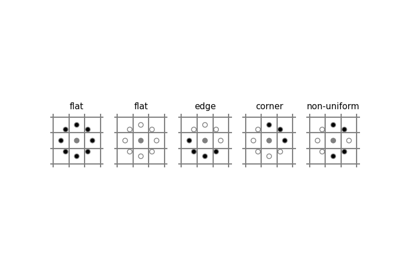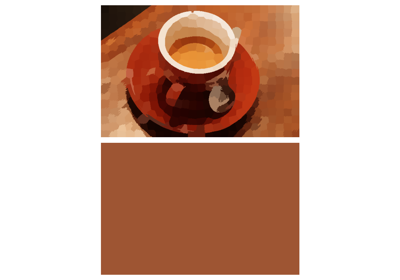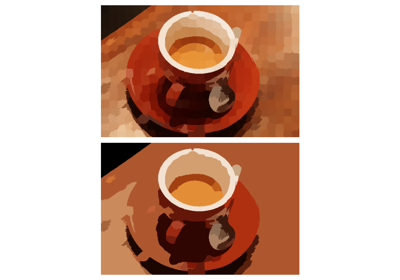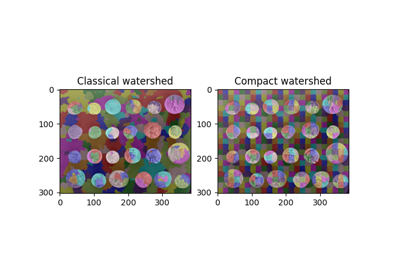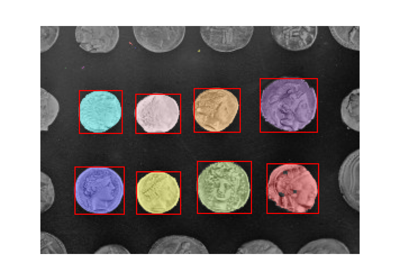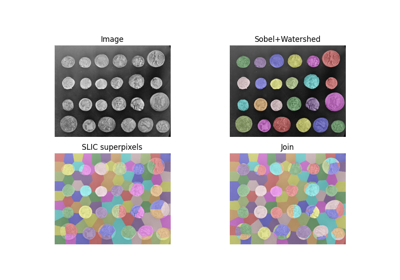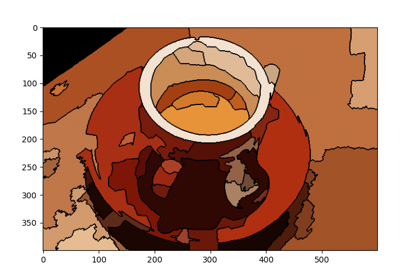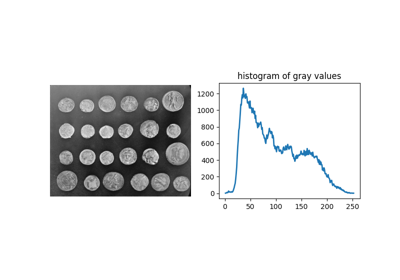## deltaE_cie76¶

skimage.color.deltaE_cie76(lab1, lab2)[source]

Euclidean distance between two points in Lab color space

Parameters
lab1array_like

reference color (Lab colorspace)

lab2array_like

comparison color (Lab colorspace)

Returns
dEarray_like

distance between colors lab1 and lab2

References

1

https://en.wikipedia.org/wiki/Color_difference

2

A. R. Robertson, “The CIE 1976 color-difference formulae,” Color Res. Appl. 2, 7-11 (1977).

## deltaE_ciede94¶

skimage.color.deltaE_ciede94(lab1, lab2, kH=1, kC=1, kL=1, k1=0.045, k2=0.015)[source]

Color difference according to CIEDE 94 standard

Accommodates perceptual non-uniformities through the use of application specific scale factors (kH, kC, kL, k1, and k2).

Parameters
lab1array_like

reference color (Lab colorspace)

lab2array_like

comparison color (Lab colorspace)

kHfloat, optional

Hue scale

kCfloat, optional

Chroma scale

kLfloat, optional

Lightness scale

k1float, optional

first scale parameter

k2float, optional

second scale parameter

Returns
dEarray_like

color difference between lab1 and lab2

Notes

deltaE_ciede94 is not symmetric with respect to lab1 and lab2. CIEDE94 defines the scales for the lightness, hue, and chroma in terms of the first color. Consequently, the first color should be regarded as the “reference” color.

kL, k1, k2 depend on the application and default to the values suggested for graphic arts

Parameter

Graphic Arts

Textiles

kL

1.000

2.000

k1

0.045

0.048

k2

0.015

0.014

References

1

https://en.wikipedia.org/wiki/Color_difference

2

http://www.brucelindbloom.com/index.html?Eqn_DeltaE_CIE94.html

## deltaE_ciede2000¶

skimage.color.deltaE_ciede2000(lab1, lab2, kL=1, kC=1, kH=1)[source]

Color difference as given by the CIEDE 2000 standard.

CIEDE 2000 is a major revision of CIDE94. The perceptual calibration is largely based on experience with automotive paint on smooth surfaces.

Parameters
lab1array_like

reference color (Lab colorspace)

lab2array_like

comparison color (Lab colorspace)

kLfloat (range), optional

lightness scale factor, 1 for “acceptably close”; 2 for “imperceptible” see deltaE_cmc

kCfloat (range), optional

chroma scale factor, usually 1

kHfloat (range), optional

hue scale factor, usually 1

Returns
deltaEarray_like

The distance between lab1 and lab2

Notes

CIEDE 2000 assumes parametric weighting factors for the lightness, chroma, and hue (kL, kC, kH respectively). These default to 1.

References

1

https://en.wikipedia.org/wiki/Color_difference

2
3

M. Melgosa, J. Quesada, and E. Hita, “Uniformity of some recent color metrics tested with an accurate color-difference tolerance dataset,” Appl. Opt. 33, 8069-8077 (1994).

## deltaE_cmc¶

skimage.color.deltaE_cmc(lab1, lab2, kL=1, kC=1)[source]

Color difference from the CMC l:c standard.

This color difference was developed by the Colour Measurement Committee (CMC) of the Society of Dyers and Colourists (United Kingdom). It is intended for use in the textile industry.

The scale factors kL, kC set the weight given to differences in lightness and chroma relative to differences in hue. The usual values are kL=2, kC=1 for “acceptability” and kL=1, kC=1 for “imperceptibility”. Colors with dE > 1 are “different” for the given scale factors.

Parameters
lab1array_like

reference color (Lab colorspace)

lab2array_like

comparison color (Lab colorspace)

Returns
dEarray_like

distance between colors lab1 and lab2

Notes

deltaE_cmc the defines the scales for the lightness, hue, and chroma in terms of the first color. Consequently deltaE_cmc(lab1, lab2) != deltaE_cmc(lab2, lab1)

References

1

https://en.wikipedia.org/wiki/Color_difference

2

http://www.brucelindbloom.com/index.html?Eqn_DeltaE_CIE94.html

3

F. J. J. Clarke, R. McDonald, and B. Rigg, “Modification to the JPC79 colour-difference formula,” J. Soc. Dyers Colour. 100, 128-132 (1984).